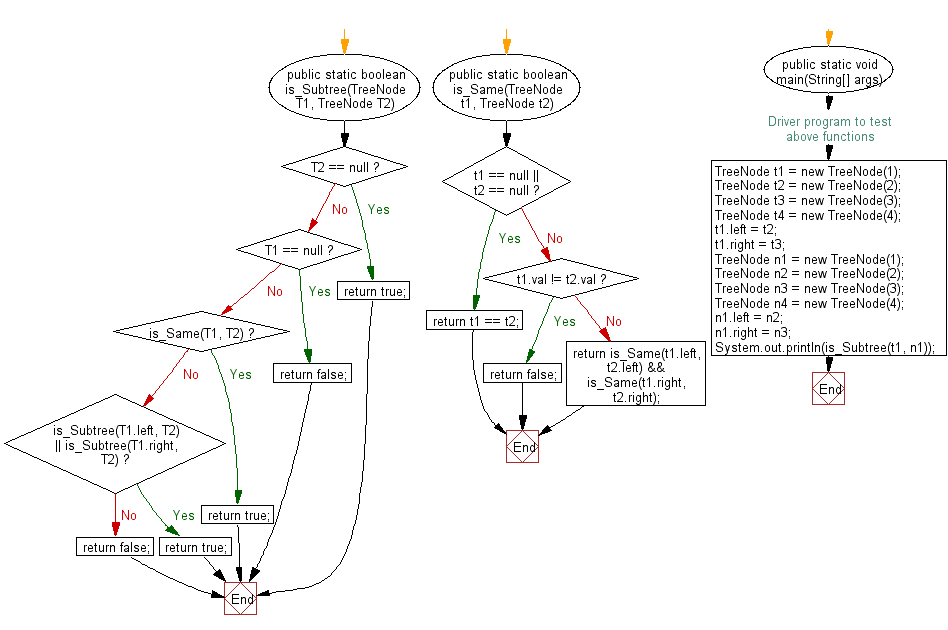﻿ Java exercises: Test if a binary tree is a subtree of another binary tree - w3resource# Java Exercises: Test if a binary tree is a subtree of another binary tree

## Java Basic: Exercise-150 with Solution

Write a Java program to test if a binary tree is a subtree of another binary tree.

Sample Solution:

Java Code:

``````public  class  Solution {
/**
* @param T1, T2: The roots of binary tree.
* @return: True if T2 is a subtree of T1, or false.
*/
public static boolean is_Subtree(TreeNode T1, TreeNode T2) {
if (T2 == null) return true;
if (T1 == null) return false;
if (is_Same(T1, T2)) return true;
if (is_Subtree(T1.left, T2) || is_Subtree(T1.right, T2)) return true;
return false;
}

public static boolean is_Same(TreeNode t1, TreeNode t2) {
if (t1 == null || t2 == null) {
return t1 == t2;
} else if (t1.val != t2.val) {
return false;
} else {
return is_Same(t1.left, t2.left) && is_Same(t1.right, t2.right);
}
}
/* Driver program to test above functions */
public static void main(String[] args) {
TreeNode t1 = new TreeNode(1);
TreeNode t2 = new TreeNode(2);
TreeNode t3 = new TreeNode(3);
TreeNode t4 = new TreeNode(4);
t1.left  = t2;
t1.right = t3;

TreeNode n1 = new TreeNode(1);
TreeNode n2 = new TreeNode(2);
TreeNode n3 = new TreeNode(3);
TreeNode n4 = new TreeNode(4);
n1.left  = n2;
n1.right = n3;

System.out.println(is_Subtree(t1, n1));
}
}
class TreeNode {
public int val;
public TreeNode left, right;

public TreeNode(int val) {
this.val = val;
this.left = this.right = null;
}
}
```
```

Sample Output:

```true
```

Flowchart:Java Code Editor:

What is the difficulty level of this exercise?

﻿

## Java: Tips of the Day

Execution of console commands:

Java allows you to execute console commands directly from code using a class Runtime. It is very important not to forget about exception handling.

For example, let's try to open a PDF file through a Java terminal:

```import java.io.BufferedReader;
import java.io.InputStream;

public class ShellCommandExec {

public static void main(String[] args) {
String gnomeOpenCommand = "gnome-open //home//user//Documents//MyDoc.pdf";

try {
Runtime rt = Runtime.getRuntime();
Process processObj = rt.exec(gnomeOpenCommand);

InputStream stdin = processObj.getErrorStream();

String myoutput = "";